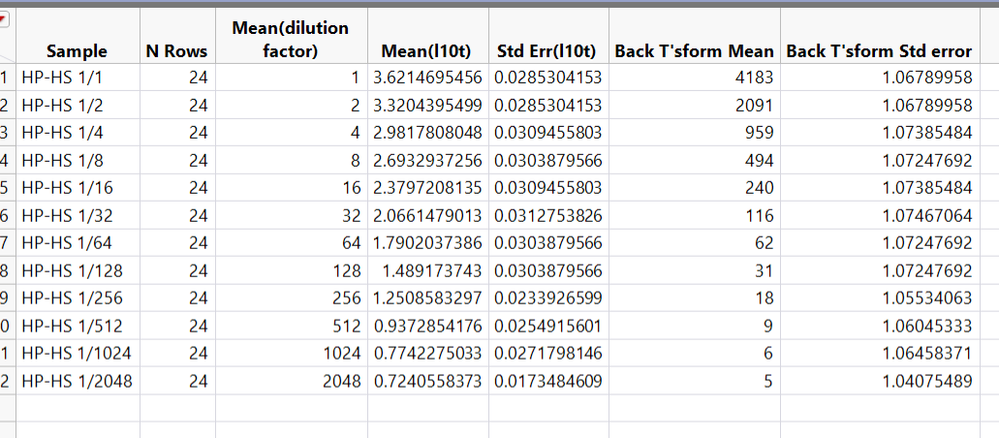Choose Language Hide Translation Bar
Highlighted

## 95% confidence intervals

Hi All,

l am trying to get a summary from a data table, l would like the mean of a variable l10t, the standard error, and 95% confidence interval. It all seemed fairly straight forward, but am having trouble with the 95% confidence intervals, have tried different ways to no avail, currently have

``````dt2<< Summary(
Group( :Sample ),
Mean( :dilution factor, :l10t ),
Freq( "None" ),
Weight( "None" ),
Output Table( "log10t" ),
Std Err( :l10t ),
Confidence Interval( :l10t, 0.95),

l am obviously doing something incorrectly, can anyone offer some advice.

Thanks Heaps

4 REPLIES 4
Highlighted

## Re: 95% confidence intervals

You can accomplish something similar like this:

``````Names Default To Here( 1 );
dt = Open( "\$SAMPLE_DATA/Big Class.jmp" );
platform = dt << Oneway(
Y( :height ),
X( :sex ),
Means and Std Dev( 1 ),
Box Plots( 1 ),
Mean Diamonds( 1 ),
Mean Error Bars( 1 ),
Std Dev Lines( 1 ),
Connect Means( 1 ),
Points Jittered( 1 ),
Grand Mean( 0 )
);
Wait( 0 );
Report( platform )[Outline Box( "Oneway Analysis of height By sex" )][
Outline Box( "Means and Std Deviations" )][Table Box( 1 )] << Make Into Data Table;
Report( platform ) << Close Window;``````

Place your group variable in the X() role.

Highlighted

## Re: 95% confidence intervals

Hi mzwald,

Thanks very much for your help and your suggestion, i tried this code

``````platform = dt2 << Oneway(
Y( :l10t ),
X( :fold dilution ),
Means and Std Dev( 1 ),
Box Plots( 1 ),
Mean Diamonds( 1 ),
Mean Error Bars( 1 ),
Std Dev Lines( 1 ),
Connect Means( 1 ),
Points Jittered( 1 ),
Grand Mean( 0 )
);
Wait( 0 );
Report( platform )[Outline Box( "Oneway Analysis of height By sex" )][
Outline Box( "Means and Std Deviations" )][Table Box( 1 )] << Make Into Data Table;
Report( platform ) << Close Window;``````

and received the following error message

``Cannot subscript Display Box in access or evaluation of 'Report(platform)[Outline Box("Oneway Analysis of height By sex")]' , Report( platform )[/*###*/Outline Box( "Oneway Analysis of height By sex" )]``

however, l am almost there as to what i want to achieve for my data table with this,

``````dt2<< Summary(
Group( :Sample ),
Mean( :dilution factor, :l10t ),
Freq( "None" ),
Weight( "None" ),
Output Table( "log10t" ),
Std Err( :l10t ),
Confidence Interval( :Name ("Mean(l10t)")),
);

Loq = Data Table( "log10t" );

Loq << New Column( "Back T'sform Mean", Numeric, Continuous, Formula( Round(10^:Name ("Mean(l10t)"),0)));

Loq << New Column( "Back T'sform Std error", Numeric, Continuous, Formula(10^:Name ("Std Err(l10t)"), Format( "fixed dec", 6, 0 ) ) );``````

giving me this,so l am almost there, l was hoping the confidence intervals would be a single simple line of code.

Thanks

Mickyboy

Highlighted

## Re: 95% confidence intervals

Well it looks like Summary doesn't currently support CI's, but you can always make a formula for them yourself.
The formulas for a 95% confidence interval (assuming a normal distribution) would be:
Upper95CI = mean + 1.96*stdev
Lower95CI = mean - 1.96*stdev
You can generate those two columns from the fields returned by the Summary platform. Those formulas will get you pretty close to what you are looking for.

Highlighted

## Re: 95% confidence intervals

Hi mzwald,

i have used the following and has worked a treat

``New Column( "Lower 95% CI", Numeric, Continuous, Formula ( Round(:Name ("Mean(l10t)") - (:Name("Std Err(l10t)") * t Quantile (0.975,23)), 4)));``

if i have used the following to create a variable

``````dt2<< Summary(
Group( :Sample ),
Mean( :dilution factor, :l10t ),
Freq( "None" ),
Weight( "None" ),
Output Table( "log10t" ),
Std Err( :l10t ),